# 力扣 (LeetCode)-合并两个有序链表,删除排序数组中的重复项,JavaScript笔记｜刷题打卡

Github来源：力扣 (LeetCode)｜刷题打卡 | 求星星 ✨ | 给个❤️关注，❤️点赞，❤️鼓励一下作者

[已开启]任务一：刷题打卡 * 10 篇

## 前言

❤️笔芯❤️~

### 21. 合并两个有序链表

``````输入：l1 = [1,2,4], l2 = [1,3,4]

• 使用递归来解，将两个链表头部较小的一个与剩下的元素合并，并返回排好序的链表头，当两条链表中的一条为空时终止递归。
• 逐一比较最小

``````/**
* function ListNode(val, next) {
*     this.val = (val===undefined ? 0 : val)
*     this.next = (next===undefined ? null : next)
* }
*/
/**
* @param {ListNode} l1
* @param {ListNode} l2
* @return {ListNode}
*/
var mergeTwoLists = function(l1, l2) {

// 创建一个空的链表
let curr = new ListNode();
// 创建一个头
let dummy = curr;

while(l1!== null && l2 !==null){
// 判断那个链表的值小，把小的连接起来
if(l1.val<l2.val){
curr.next = l1;
l1 = l1.next;
}else{
curr.next = l2;
l2= l2.next
}
curr = curr.next;
}

if(l1!==null){
curr.next = l1;
}
if(l2!==null){
curr.next = l2;
}
return dummy.next;
};

`````` */
/**
* @param {ListNode} l1
* @param {ListNode} l2
* @return {ListNode}
*/
const mergeTwoLists = function (l1, l2) {
if (l1 === null) {
return l2;
}
if (l2 === null) {
return l1;
}
if (l1.val < l2.val) {
l1.next = mergeTwoLists(l1.next, l2);
return l1;
} else {
l2.next = mergeTwoLists(l1, l2.next);
return l2;
}
};

### JavaScript笔记

1. 变量

JavaScript的类型有数字，字符串，布尔值，函数和对象，还有`undefined``null`，数组，日期，正则表达式。

`JavaScript`中，使用关键字`var`，而不必指定变量类型，所以，`JavaScript`不是强类型语言。

1. `typeof`操作符返回变量或表达式的类型
``````console.log(typeof num); // number
console.log(typeof 'jeskson'); // string
console.log(typeof true); // boolean
console.log(typeof [1,2,3]); // object
console.log(typeof {name:'jeskson'}); // object

1. `JavaScript`支持`delete`操作符，可以删除对象里的属性
``````var my = {name: 'jeskson', age: 1};
delete my.age;
console.log(my); // {name: 'jeskson'}

1. 真假
``````nudefined // false

null // false

1. `JavaScript`面向对象编程
• a. `var obj = new Object();`
• b. `var obj = {}`
``````obj = {
name: 'jeskson'
age: 1
};

1. `ECMAScript`是一种脚本语言规范，`JavaScript`是这个规范的一个实现。

### `ECMAScript6`的功能

1. `let``const`
2. 模板字面量
3. 解构
4. 展开操作符
5. 箭头函数`=>`

`ES6`引入了一个`let`关键字，可以直接把`var`关键字都替换成`let``ES6`还引入了`const`关键字`，区别于`const`定义的变量是只读的，也就是常量。

``````let circleArea = (r) => 3.14 * r * r;

``````function sum (x = 1, y = 2, z = 3) {
return x + y + z
};
console.log(sum(4,2)); //输出9

• `ES5`中，我们可以用`apply()`函数把数组转化为参数
• `ES6`有了展开操作符（`...`
• 在函数中，展开操作符（`...`）也可以代替`arguments`，当作剩余参数使用
``````console.log(sum(...params));

console.log(sum.apply(undefined, params));

function da(x, y, ...a) {
return (x + y) * a.length;
}

function da(x, y) {
var a = Array.prototype.slice.call(arguments, 2);
return (x + y) * a.length;
}

• 可以用来一次初始化多个变量
• 可以用来进行值的互换，而不需要创建临时变量

``````var [x, y] = ['a', 'b'];
var obj = { x, y };
console.log(obj); // { x: "a", y: "b" }

``````var hello = {
printHello() {
console.log('Hello');
}
}

var hello = {
printHello: function printHello() {
console.log('Hello');
}
};

``````function dada(name, age) {
this.name = name;
this.age = age;
}
console.log(this.name);
};

// 声明一个有constructor函数
constructor(name, age) {
this.name = name;
this.age = age;
}
printWork() {
console.log(this.name);
}
}

### 继承

`JavaScript`面向对象编程是基于原型实现的

``````class itBook extends Book {
costructor(title, pages, isbn, technology) {
super(title, pages, isbn);
this.technology = technology;
}
printTechnology() {
console.log(this.technology);
}
}

let daBook = new itBook('魔王哪吒算法', '222', '1333', 'JavaScript');

### 使用属性存取器

``````class Person {
constructor (name) {
this._name = name;
}
get name() {
return this._name;
}
set name(value) {
this._name = value;
}
}

### 26. 删除排序数组中的重复项

``````示例 1:

``````// nums 是以“引用”方式传递的。也就是说，不对实参做任何拷贝
int len = removeDuplicates(nums);

// 在函数里修改输入数组对于调用者是可见的。
// 根据你的函数返回的长度, 它会打印出数组中该长度范围内的所有元素。
for (int i = 0; i < len; i++) {
print(nums[i]);
}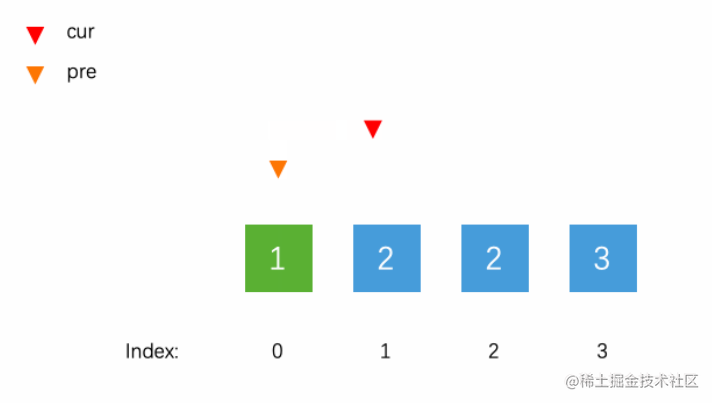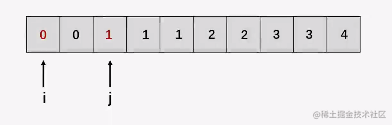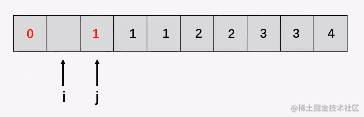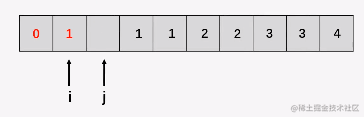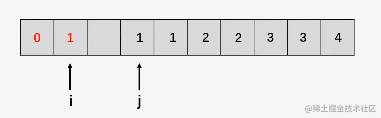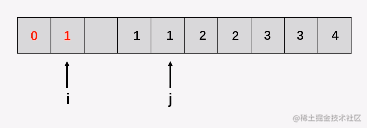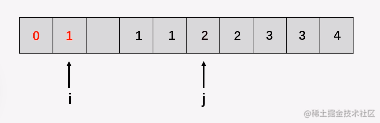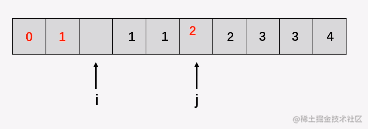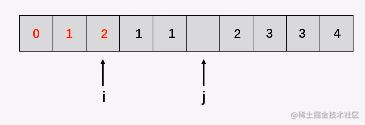``````/**
* @param {number[]} nums
* @return {number}
*/
var removeDuplicates = function (nums) {
if (size == 0) return 0;
let slowP = 0;
for (let fastP = 0; fastP < nums.length; fastP++) {
if (nums[fastP] !== nums[slowP]) {
slowP++;
nums[slowP] = nums[fastP];
}
}
return slowP + 1;
};

### 回看笔者往期高赞文章，也许能收获更多喔！

❤️关注+点赞+收藏+评论+转发❤️，原创不易，鼓励笔者创作更好的文章

### 我们下期见！

`github`收录，欢迎`Star`github.com/webVueBlog/…